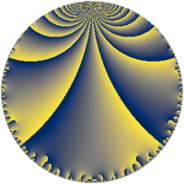# Properties

 Label 33.6.fLevel $33$ Weight $6$ Character orbit 33.f Rep. character $\chi_{33}(2,\cdot)$ Character field $\Q(\zeta_{10})$ Dimension $72$ Newform subspaces $1$ Sturm bound $24$ Trace bound $0$

# Related objects

## Defining parameters

 Level: $$N$$ $$=$$ $$33 = 3 \cdot 11$$ Weight: $$k$$ $$=$$ $$6$$ Character orbit: $$[\chi]$$ $$=$$ 33.f (of order $$10$$ and degree $$4$$) Character conductor: $$\operatorname{cond}(\chi)$$ $$=$$ $$33$$ Character field: $$\Q(\zeta_{10})$$ Newform subspaces: $$1$$ Sturm bound: $$24$$ Trace bound: $$0$$

## Dimensions

The following table gives the dimensions of various subspaces of $$M_{6}(33, [\chi])$$.

Total New Old
Modular forms 88 88 0
Cusp forms 72 72 0
Eisenstein series 16 16 0

## Trace form

 $$72q + 18q^{3} - 262q^{4} + 15q^{6} - 10q^{7} + 292q^{9} + O(q^{10})$$ $$72q + 18q^{3} - 262q^{4} + 15q^{6} - 10q^{7} + 292q^{9} + 1854q^{12} - 10q^{13} - 762q^{15} - 10122q^{16} + 4815q^{18} + 4460q^{19} + 4628q^{22} - 805q^{24} + 13708q^{25} + 6108q^{27} - 28130q^{28} - 15470q^{30} + 4340q^{31} - 508q^{33} + 18732q^{34} - 56461q^{36} + 978q^{37} + 23360q^{39} + 69750q^{40} + 60788q^{42} - 31356q^{45} - 52090q^{46} + 4238q^{48} - 58448q^{49} - 178950q^{51} - 14190q^{52} + 86600q^{55} + 266190q^{57} + 137102q^{58} + 284090q^{60} - 77890q^{61} - 120330q^{63} - 379114q^{64} - 323304q^{66} + 42668q^{67} - 271816q^{69} + 87176q^{70} + 343960q^{72} + 116440q^{73} + 326202q^{75} + 155512q^{78} - 350590q^{79} - 208088q^{81} - 606424q^{82} - 220680q^{84} + 665610q^{85} + 1152974q^{88} + 293440q^{90} + 621014q^{91} + 478456q^{93} - 521270q^{94} - 1246430q^{96} - 1030446q^{97} - 590000q^{99} + O(q^{100})$$

## Decomposition of $$S_{6}^{\mathrm{new}}(33, [\chi])$$ into newform subspaces

Label Dim. $$A$$ Field CM Traces $q$-expansion
$$a_2$$ $$a_3$$ $$a_5$$ $$a_7$$
33.6.f.a $$72$$ $$5.293$$ None $$0$$ $$18$$ $$0$$ $$-10$$